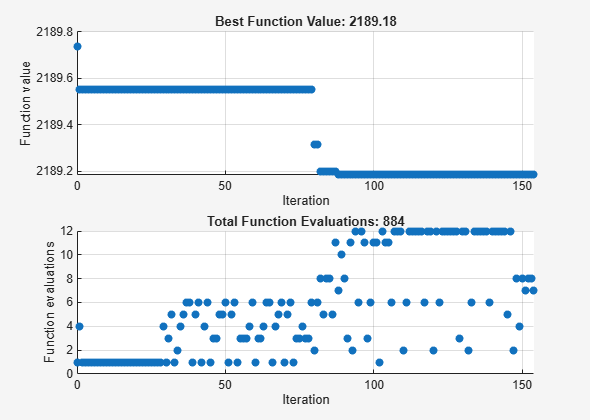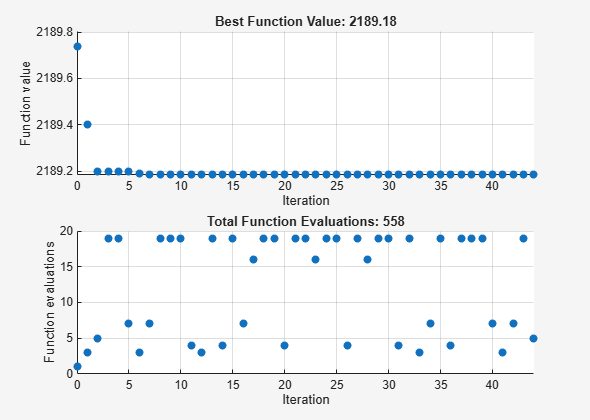Documentation

## Effects of Some Pattern Search Options

This example shows the effects of some options for pattern search. The options include plotting, stopping criteria, and other algorithmic controls for speeding a solution.

### Set Up a Problem for Pattern Search

The problem to minimize is a quadratic function of six variables subect to linear equality and inequality constraints. The objective function, `lincontest7`, is included with Global Optimization Toolbox software.

`type lincontest7`
```function y = lincontest7(x); %LINCONTEST7 objective function. % y = LINCONTEST7(X) evaluates y for the input X. Make sure that x is a column % vector, whereas objective function gets a row vector. % Copyright 2003-2004 The MathWorks, Inc. x = x'; %Define a quadratic problem in terms of H and f (From web unknown source) H = [36 17 19 12 8 15; 17 33 18 11 7 14; 19 18 43 13 8 16; 12 11 13 18 6 11; 8 7 8 6 9 8; 15 14 16 11 8 29]; f = [ 20 15 21 18 29 24 ]'; y = 0.5*x'*H*x + f'*x; ```

Specify the function handle `@lincontest7` as the objective function.

`objectiveFcn = @lincontest7;`

The objective function accepts a row vector of length six. Specify an initial point for the optimization.

`x0 = [2 1 0 9 1 0];`

Create linear constraint matrices representing the constraints `Aineq*x <= Bineq` and `Aeq*x = Beq`. For details, see Linear Constraints (Optimization Toolbox).

```Aineq = [-8 7 3 -4 9 0 ]; Bineq = ; Aeq = [7 1 8 3 3 3; 5 0 5 1 5 8; 2 6 7 1 1 8; 1 0 0 0 0 0]; Beq = [84 62 65 1];```

Run the `patternsearch` solver, and observe how many iterations and function evaluations it takes to arrive at the solution.

`[X1,Fval,Exitflag,Output] = patternsearch(objectiveFcn,x0,Aineq,Bineq,Aeq,Beq);`
```Optimization terminated: mesh size less than options.MeshTolerance. ```
`fprintf('The number of iterations was : %d\n', Output.iterations);`
```The number of iterations was : 244 ```
`fprintf('The number of function evaluations was : %d\n', Output.funccount);`
```The number of function evaluations was : 1895 ```
`fprintf('The best function value found was : %g\n', Fval);`
```The best function value found was : 2189.03 ```

### Adding Visualization

Monitor the optimization process by specifying two plot functions. Specify the plot functions as the `PlotFcn` option by using `optimoptions`. One plot function, `psplotbestf`, plots the best objective function value at every iteration. Another plot function, `psplotfuncount`, plots the number of times the objective function is evaluated at each iteration. Set these two plot functions in a cell array.

`opts = optimoptions(@patternsearch,'PlotFcn',{@psplotbestf,@psplotfuncount});`

Run the `patternsearch` solver. Since there are no upper or lower bound constraints and no nonlinear constraints, pass empty arrays (`[]`) for the seventh, eighth, and ninth arguments.

```[X1,Fval,ExitFlag,Output] = patternsearch(objectiveFcn,x0,Aineq,Bineq, ... Aeq,Beq,[],[],[],opts);``````Optimization terminated: mesh size less than options.MeshTolerance. ```

### Mesh Parameters

Initial mesh size

The pattern search algorithm uses a set of rational basis vectors to generate search directions. It performs a search along the search directions using the current mesh size. The solver starts with an initial mesh size of `1` by default. To start the initial mesh size at `10`, set the `InitialMeshSize` option:

`options = optimoptions(opts,'InitialMeshSize',10);`

Mesh scaling

A mesh can be scaled to improve the minimization of a badly scaled optimization problem. Scale is used to rotate the pattern by some degree and scale along the search directions. The scale option is on (`true`) by default but can be turned off if the problem is well scaled. In general, if the problem is badly scaled, setting this option to `true` may help in reducing the number of function evaluations. For this objective function, set `ScaleMesh` to `false`, because `lincontest7` is a well-scaled objective function.

`opts = optimoptions(opts,'ScaleMesh',false);`

Mesh accelerator

Direct search methods require many function evaluations as compared to derivative-based optimization methods. The pattern search algorithm can quickly find the neighborhood of an optimum point, but can be slow in detecting the minimum itself. This is the cost of not using derivatives. The `patternsearch` solver can reduce the number of function evaluations using an accelerator. When the accelerator is on (`opts.AccelerateMesh = true`) the mesh size is contracted rapidly after some minimum mesh size is reached. This option is recommended only for smooth problems, otherwise you can lose some accuracy. The accelerator is off (`false`) by default. Here, set the `AccelerateMesh` to `true` because the objective function is smooth.

`opts = optimoptions(opts,'AccelerateMesh',true);`

Run the `patternsearch` solver.

```[X2,Fval,ExitFlag,Output] = patternsearch(objectiveFcn,x0,Aineq,Bineq, ... Aeq,Beq,[],[],[],opts);``````Optimization terminated: mesh size less than options.MeshTolerance. ```
`fprintf('The number of iterations was : %d\n', Output.iterations);`
```The number of iterations was : 200 ```
`fprintf('The number of function evaluations was : %d\n', Output.funccount);`
```The number of function evaluations was : 1327 ```
`fprintf('The best function value found was : %g\n', Fval);`
```The best function value found was : 2189.03 ```

These option setting reduce the number of iterations and the number of function evaluations, and there is no apparent loss of accuracy.

### Stopping Criteria and Tolerances

What are MeshTolerance, StepTolerance and FunctionTolerance?

`MeshTolerance` is a tolerance on the mesh size. If the mesh size is less than `MeshTolerance`, the solver stops. `StepTolerance` is used as the minimum tolerance on the change in the current point to the next point. `FunctionTolerance` is used as the minimum tolerance on the change in the function value from the current point to the next point.

Set the `MeshTolerance` to 1e-7, which is ten times smaller than the default value. This setting can increase the number of function evaluations and iterations, and can lead to a more accurate solution.

`opts.MeshTolerance = 1e-7;`

### Search Methods in Pattern Search

The pattern search algorithm can use an additional search at every iteration. This option is called `SearchFcn`. When you set a `SearchFcn`, that search is done first before the mesh search. If the `SearchFcn` is successful, `patternsearch` skips the mesh search, commonly called the `PollFcn`, for that iteration. If the search method is unsuccessful in improving the current point, `patternsearch` performs the poll.

There are five different search method options. These search methods include `searchga` and `searchneldermead`, which are two different optimization algorithms. It is recommended to use these methods only for the first iteration, which is the default. Using these methods repeatedly at every iteration might not improve the results and could be computationally expensive. One the other hand, the `searchlhs`, which generates Latin hypercube points, can be used in every iteration or possibly every 10 iterations. Other choices for search methods include Poll methods such as positive basis N+1 or positive basis 2N.

A recommended strategy is to use the positive basis N+1 (which requires at most N+1 points to create a pattern) as a search method and positive basis 2N (which requires 2N points to create a pattern) as a poll method. Update the options structure to use `positivebasisnp1` as the search method. Since the positive basis 2N is the default `PollFcn`, do not set that option.

`opts.SearchFcn = @positivebasisnp1;`

Run the `patternsearch` solver.

```[X5,Fval,ExitFlag,Output] = patternsearch(objectiveFcn,x0,Aineq,Bineq,Aeq,Beq, ... [],[],[],opts);``````Optimization terminated: mesh size less than options.MeshTolerance. ```
`fprintf('The number of iterations was : %d\n', Output.iterations);`
```The number of iterations was : 70 ```
`fprintf('The number of function evaluations was : %d\n', Output.funccount);`
```The number of function evaluations was : 867 ```
`fprintf('The best function value found was : %g\n', Fval);`
```The best function value found was : 2189.03 ```

With these options, the total number of iterations and function evaluations decrease. This decrease occurs even though the mesh tolerance is smaller than the previous value, and, as you can see in the exit messages, the mesh tolerance is the stopping criterion that halts the solver.

Watch now